## 3.18Second order time schemes

A Taylor’s series expansion between the current timeand ‘old’ time at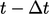relates to the Euler implicit scheme by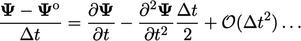(3.25)
The truncation error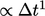, i.e. it is ﬁrst order accurate in time when the time derivative relates to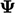at the current time, as shown in the ﬁgure below. Despite its low order, the Euler scheme is suﬃciently accurate for many simulations since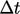is generally small when it corresponds to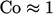.

Nevertheless, a second order time scheme may be required for simulations that demand higher temporal accuracy or to enable greater computational eﬃciency by running with larger.

### Backward scheme

In Eq. (3.25 ) we can replace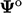by the values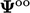at ‘old-old’ time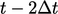. Subtracting the expression from Eq. (3.25 ) and rearranging terms gives the following relation for the second derivative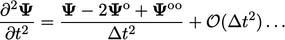(3.26)
Substituting Eq. (3.26 ) into Eq. (3.25) gives the backward scheme which is second order accurate, using values at three time levels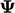,and: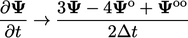(3.27)

### Crank-Nicolson scheme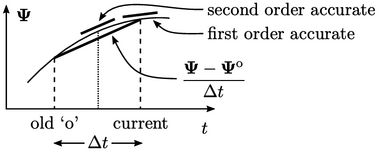An implicit solution expresses the terms in an equation, e.g. advection, Laplacian, at the current time. The Crank-Nicolson method,13 expresses the terms at the midpoint between the current and old times, to make the Euler time scheme second order accurate. Denoting discretised terms except the time derivative by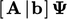, the Crank-Nicolson method solves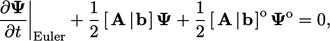(3.28)
where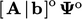is calculated using old time values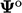.

A modern version of the scheme replaces the two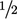factors by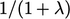and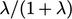, introducing the ‘oﬀset coeﬃcient’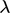, where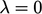corresponds to Euler implicit and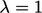is Crank-Nicolson Eq. (3.28 ). If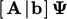is discretised implicitly (as normal), the Crank-Nicolson scheme can be represented as a time derivative discretised by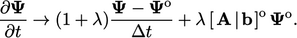(3.29)
In practice,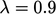is generally used to ensure solution stability.
13John Crank and Phyllis Nicolson, A practical method for numerical evaluation of solutions of partial diﬀerential equations of the heat conduction type, 1947.

Notes on CFD: General Principles - 3.18 Second order time schemes